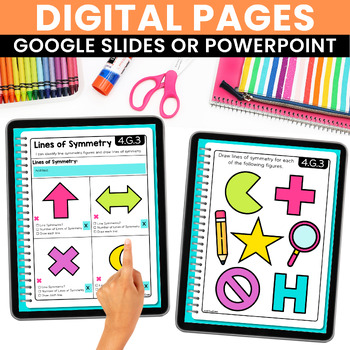DID YOU KNOW:
Seamlessly assign resources as digital activities

Learn how in 5 minutes with a tutorial resource. Try it Now

Learn More# Math Interactive Notebook 4th Grade Geometry3rd - 5th, Homeschool
Subjects
Standards
Resource Type
Formats Included
• PDF
Pages
23 pages

#### Also included in

1. What are Interactive Math Notebooks?Interactive math notebooks are teaching pages used to introduce and teach math concepts and skills. Each set of notebook pages includes definitions, explanations, rules, and algorithms that may be needed to address each standard. Practice problems are included to
\$25.50
\$31.50
Save \$6.00
2. This BUNDLE includes everything you need for 4th grade math!!★ Interactive Notebook Pages★ Math Quick Checks ★ Math Menus (Perfect for homework or early finishers!!)★ Open-Ended Math Pages★ Math Mastery Task Cards★ Units for Fractions, Decimals, Measurement & GeometryFeel free to email with any
\$82.50
\$102.70
Save \$20.20

### Description

What are Interactive Math Notebooks?

Interactive math notebooks are teaching pages used to introduce and teach math concepts and skills. Each set of notebook pages includes definitions, explanations, rules, and algorithms that may be needed to address each standard. Practice problems are included to be used for guided practice. Once completed, these pages serve as a great reference tool for students to refer back throughout the year.

How do I use them with my students?

Notebook pages can be used to introduce, reinforce, or review math standards. They can be used for direct instruction for a whole class or in small groups. For the printable version, students first cut out the pages and glue them into a spiral notebook. With teacher guidance, students take notes and work out practice problems. Students can then highlight or add color to their notebook pages. The digital pages include the same content, but require no cutting or pasting!

What materials are needed for printable notebook pages?

A spiral notebook for each student, scissors, glue sticks, pencils, and colored pencils, crayons, markers (optional)

What is included in this resource?

• Notebook pages for each standard. Each concept or skill includes a double-page spread. (Printable & Digital)
• Answer Key (Available with printable pages)

What standards are covered?

• Points, Lines, Line Segments, and Rays (4.G.1)
• Angles (right, acute, obtuse (4.G.1)
• Perpendicular and Parallel Lines (4.G.1)
• Mystery Shape Search (4.G.2)
• Lines of Symmetry (4.G.3)

★★This resource is included in myULTIMATE 4th Grade Math Bundle!!★★

Check out the rest of my Math Activities for 4th Grade:

4th Grade Interactive Math Notebook: OA

4th Grade Interactive Math Notebook: NBT

4th Grade Interactive Math Notebook: NF

4th Grade Interactive Math Notebook: MD

4th Grade Interactive Math Notebook: G

Common Core Math Quick Checks

Math Exit Slips

Solve & Sort Math Centers

Total Pages
23 pages
N/A
Teaching Duration
2 Weeks
Report this Resource to TpT
Reported resources will be reviewed by our team. Report this resource to let us know if this resource violates TpT’s content guidelines.

### Standards

to see state-specific standards (only available in the US).
Recognize a line of symmetry for a two-dimensional figure as a line across the figure such that the figure can be folded along the line into matching parts. Identify line-symmetric figures and draw lines of symmetry.
Classify two-dimensional figures based on the presence or absence of parallel or perpendicular lines, or the presence or absence of angles of a specified size. Recognize right triangles as a category, and identify right triangles.
Draw points, lines, line segments, rays, angles (right, acute, obtuse), and perpendicular and parallel lines. Identify these in two-dimensional figures.